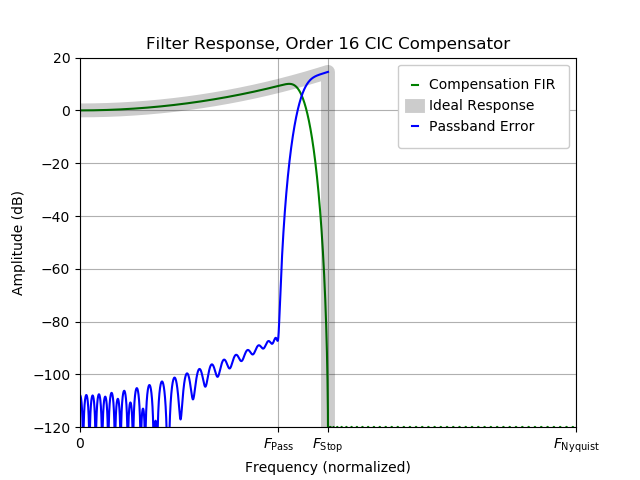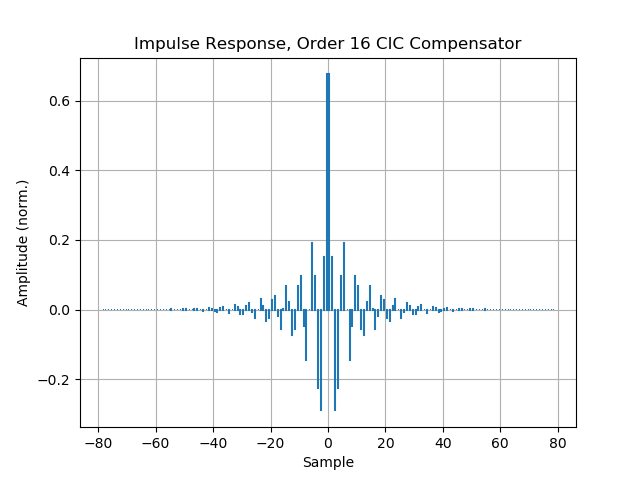# Designing CIC Compensation Filters

This page describes how to design CIC compensation filters using open-source tools. Due to the use of newer algorithms, compensators designed using this method should outperform MATLAB's built-in "ciccomp" filters.

## A Little Motivation

Occasionally, DSP designers need to build decimators.Here, the goal is to remove high-frequency content from a sampled data source, producing a new sequence of data samples at a lower sampling rate.

From a theoretical perspective, the canonical approach to decimation is to use a Finite Impulse Response (FIR) filter. FIR decimators are well-understood, well-behaved, and approachable. FIR design is mature, and there exists a canonical FIR design tool (the [Parks-McClellan] or Remez algorithm) that makes it straightforward to turn a filter specification into a set of FIR coefficients. Implementations of this algorithm are available in Python/Scipy and MATLAB. More recent work has produced variations on the algorithm with better convergence [Filip].

From a practical perspective, a naive implementation of a FIR decimator (that is, a FIR followed by a decimation stage) is computationally inefficient. Here, too, there's an obvious path to follow. Polyphase techniques [Vaidyanathan] can be used to decompose a single, large FIR into a set of parallel FIRs operating at a reduced data rate.

As flexible and useful as FIR decimators are, there are a few corner cases where they don't produce adequate designs:

• When the decimation ratios involved are very high, the number of FIR coefficients needed to meet performance requirements grows. Even if the Parks-McClellan algorithm does converge to an usable FIR, the resulting coefficient vector may be impractical to use even after polyphase decomposition.
• When memory (coefficient and signal buffers) is very highly constrained (i.e. in an FPGA, or when processing many signals in a design.) The polyphase decomposition reduces the computational burden associated with the FIR, but does nothing for the amount of memory required.
• When an FIR does not offer adequate flexibility (see, for example, the variable-sampling-rate trick described below.)

In these situations, a CIC is an interesting alternative.

## Introducing the CIC Filter

A CIC, or Cascaded Integrator-Comb, is a filter that's much more restrictive than an FIR. It has a very wide transition region and is only really effective as an anti-aliasing filter for very narrowband signals. However, it's computationally extremely efficient (it requires only adders and registers, and relatively few of each) and its shortcomings can be corrected. When paired with a conventional FIR, the resulting compound decimator plays to the strengths of each.The original CIC paper [Hogenauer] is among my favourite DSP references. It is well written and approachable, and revolves around a single mathematical trick so filthy it's genuinely worth celebrating. Please stop here and go find read the original paper.

Assuming you have skimmed the original CIC paper, I will now pull out several tidbits. (Note that I'm focused on decimators, here, but there is always a dual development that applies equally to interpolators.)

In the following, we assume the compound decimation structure shown above, in which large decimation factors are dealt with by the CIC. The FIR exists only to compensate or "clean up" passband droop imposed by the CIC, and decimates by a final factor of 2 because it is convenient and efficient to do so.

## A Magic Trick: Small-Angle Approximation

The amplitude response for a CIC decimator is (using the notation from [Hogenauer]):

$$A(f) = \left[ \frac{\sin (\pi M f)}{\sin \left( \frac{\pi f}{R}\right)} \right]^N$$

The terms in this equation are defined as follows:

• $$N$$ is the number of stages in the CIC filter,
• $$M$$ is a differential delay (a tunable parameter, though I only ever use 1),
• $$R$$ is the CIC's rate-change parameter, and
• $$f$$, here, is a normalized frequency in which 0.5 corresponds to the Nyquist rate.

Hogenauer notes the small-angle approximation for the denominator term:

$$\newcommand{\sinc}{\mathop{\rm sinc}\nolimits} A(f) \approx \left[ RM \right]^N \sinc^N(\pi M f)$$

where $$\sinc(x) = \sin (\pi x)/(\pi x)$$.

Let's assume our compensation filter will only correct the frequency-dependent portions of this expression. (When $$[RM]^N$$ is a power of 2, as they are in my case, these gains are typically corrected using bit shifts, which are free in an FPGA.) We are left with the frequency-dependent portion of the expression

$$\sinc^N \left( \pi M f \right)$$

To correct for this amplitude droop, the FIR must impose the inverse gain on its passband:

$$C(f) = \sinc^{-N}(\pi Mf)$$

for $$f$$ over a passband extending as high as the normalized frequency 1/4 (that is, the Nyquist rate at the FIR's output, remembering that it is also has a decimation-by-2 rate change function.) In reality, the filter's passband edge must be relaxed below the Nyquist rate to introduce a transition region, allowing the filter's design to be tractable.

The decimation rate R does not appear in this expression! That is, provided the small-angle approximation is appropriate, a single single, fixed compensation FIR will suffice for any CIC decimation rate. This provides an easy way to build a signal path that's adaptable to multiple operating scenarios without being redesigned each time.

We have motivated the need for a compensating filter, and proposed a structure for it. We have also calculated the required passband amplitude to correct for droop in the CIC's passband. We are finally ready to calculate it.

## Compensating CIC Filters

Of the dominant signal-processing packages, unfortunately, only MATLAB provides the necessary machinery to create a CIC compensator FIR "out-of-the-box". In the past, I have managed to scrounge up a MATLAB installation in the rare case I need it. However, my lab shifted wholesale to Python a decade ago, and I'm otherwise completely MATLAB-free. Sorry, Mathworks, it's time to move on.

Here are a few open-source Parks-McClellan or Remez implementations:

Unfortunately, none of these implementations is suitable on its own. The Liquid SDR implementation allows a callback to inject the desired amplitude responses $$C(f)$$; however, this implementation did not converge well enough for me to use it. Where other implementations of the Parks-McClellan algorithm admitted a stepwise or piecewise-linear approximation to $$C(f)$$, experiments with this approach were far too brittle to produce usable results.

I was, however, able to add a callback-enabled version of Silviu-Ioan Filip's code.

## The Solution

I began with the source code here:

This code contains three implementations of an extended Parks-McClellan algorithm: two using underlying C types (double and long double), and one using an arbitrary-precision library (Pavel Holoborodko's excellent C++ wrappers for the also excellent GNU MPFR library.) I opted for the third, since I am more interested in limiting developer commitment than maximizing computational efficiency.

(As an aside, this code would benefit greatly from some refactoring by an experienced C++ developer.)

My modifications are contained in a new repository here:Enjoy!